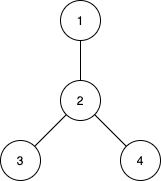# 统计子树中城市之间最大距离

## 1617. 统计子树中城市之间最大距离 (Hard)```输入：n = 4, edges = [[1,2],[2,3],[2,4]]

```

```输入：n = 2, edges = [[1,2]]

```

```输入：n = 3, edges = [[1,2],[2,3]]

```

• `2 <= n <= 15`
• `edges.length == n-1`
• `edges[i].length == 2`
• `1 <= ui, vi <= n`
• 题目保证 `(ui, vi)` 所表示的边互不相同。

[回溯算法]